• 已知十个经纬度怎么来判断一个经纬度确定）距离这十个经纬度最近的经纬度 或者判断其中一个经纬度确定）距离这十个经纬度连接起来的路径最近的距离java-ee 地图 经纬度
• ## SIFT解析（二）特征点位置确定

千次阅读 热门讨论 2013-05-27 23:07:35
确实，在图像特征识别领域，SIFT的出现是...上一篇文章，解析了SIFT特征提取的第一步高斯金字塔的构建，并详细分析了高斯金字塔以及差分高斯金字塔如何完成一个连续的尺度空间的构建。构建高斯金字塔不是目的，目的是如
最近微博上有人发起投票那篇论文是自己最受益匪浅的论文，不少人说是lowe的这篇介绍SIFT的论文。确实，在图像特征识别领域，SIFT的出现是具有重大意义的，SIFT特征以其稳定的存在，较高的区分度推进了诸多领域的发展，比如识别和配准。上一篇文章，解析了SIFT特征提取的第一步高斯金字塔的构建，并详细分析了高斯金字塔以及差分高斯金字塔如何完成一个连续的尺度空间的构建。构建高斯金字塔不是目的，目的是如何利用高斯金字塔找到极值点。
lowe在论文中阐述了为什么使用差分高斯金字塔：
1）差分高斯图像可以直接由高斯图像相减获得，简单高效
2） 差分高斯函数是尺度规范化的高斯拉普拉斯函数的近似，而高斯拉普拉斯函数的极大值和极小值点是一种非常稳定的特征点（与梯度特征、Hessian特征和Harris角点相比）
有了这些基础，我们就可以放开手脚从差分高斯金字塔中找点了。
特征点的确定主要包括两个过程：确定潜在特征点，精确确定特征点的位置和去除不稳定特征点。
确定潜在特征点
上文已经阐述，高斯拉普拉斯函数的极大值和极小值点是一种非常稳定的特征点，因此我们从差分高斯金字塔中寻找这些潜在特征点。差分高斯金字塔是一个三维空间（平面图像二维，尺度一维），因此我们在三维空间中在寻找极大值点和极小值点。具体方法是比较当前特征点的灰度值和其他26个点的灰度值的大小，这26个点包括：当前尺度下该点的8邻域以及前一尺度和后一尺度下与该点最近的9个点（9*2+8=26），如下图所示：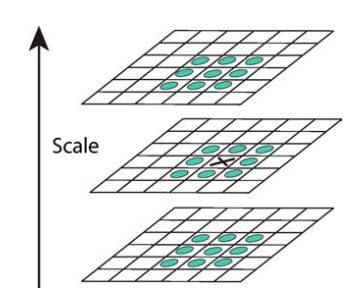OpenCV该部分源码：

void SIFT::findScaleSpaceExtrema( const vector<Mat>& gauss_pyr, const vector<Mat>& dog_pyr,
vector<KeyPoint>& keypoints ) const
{
......
for( int o = 0; o < nOctaves; o++ )//每一个八度
for( int i = 1; i <= nOctaveLayers; i++ )//对八度中的存在具有第1至第nOctaveLayers层高斯差分图像提取特征点
{
......
for( int r = SIFT_IMG_BORDER; r < rows-SIFT_IMG_BORDER; r++)//图像二维空间.行
{
......
for( int c = SIFT_IMG_BORDER; c < cols-SIFT_IMG_BORDER; c++)//图像二维空间.列
{
.......
// 当前点与26个点比较，比较两次，分别确定是否是极大值，是否是极小值
if( std::abs(val) > threshold &&
((val > 0 && val >= currptr[c-1] && val >= currptr[c+1] &&
val >= currptr[c-step-1] && val >= currptr[c-step] && val >= currptr[c-step+1] &&
val >= currptr[c+step-1] && val >= currptr[c+step] && val >= currptr[c+step+1] &&
val >= nextptr[c] && val >= nextptr[c-1] && val >= nextptr[c+1] &&
val >= nextptr[c-step-1] && val >= nextptr[c-step] && val >= nextptr[c-step+1] &&
val >= nextptr[c+step-1] && val >= nextptr[c+step] && val >= nextptr[c+step+1] &&
val >= prevptr[c] && val >= prevptr[c-1] && val >= prevptr[c+1] &&
val >= prevptr[c-step-1] && val >= prevptr[c-step] && val >= prevptr[c-step+1] &&
val >= prevptr[c+step-1] && val >= prevptr[c+step] && val >= prevptr[c+step+1]) ||
(val < 0 && val <= currptr[c-1] && val <= currptr[c+1] &&
val <= currptr[c-step-1] && val <= currptr[c-step] && val <= currptr[c-step+1] &&
val <= currptr[c+step-1] && val <= currptr[c+step] && val <= currptr[c+step+1] &&
val <= nextptr[c] && val <= nextptr[c-1] && val <= nextptr[c+1] &&
val <= nextptr[c-step-1] && val <= nextptr[c-step] && val <= nextptr[c-step+1] &&
val <= nextptr[c+step-1] && val <= nextptr[c+step] && val <= nextptr[c+step+1] &&
val <= prevptr[c] && val <= prevptr[c-1] && val <= prevptr[c+1] &&
val <= prevptr[c-step-1] && val <= prevptr[c-step] && val <= prevptr[c-step+1] &&
val <= prevptr[c+step-1] && val <= prevptr[c+step] && val <= prevptr[c+step+1])))
{
......

}
}
}
}
}

尺度空间中的极值点已经确定出来了，下面有两个问题需要解决：
（1）这些点是最终我们确定的SIFT特征点集的超集，该超集里包含许多“间谍”-----不稳定的特征点，因此必须去掉这些不稳定的特征点。这些不稳定的特征点主要包含两类：低对比度的点（对噪声敏感）和边缘点。
（2）这一步骤中极值点的坐标还是离散的整数值，如何精确确定特征点的位置。
精确确定特征点的位置：
由于图像是一个离散的空间，特征点的位置的坐标都是整数，但是极值点的坐标并不一定就是整数，如下图所示。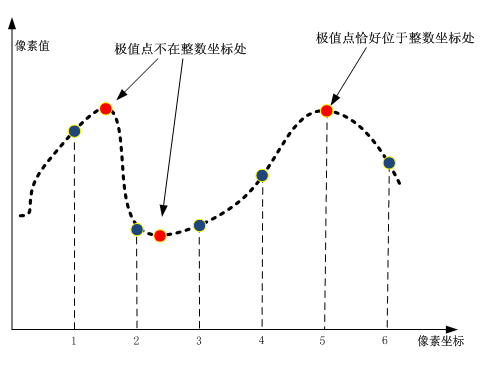因此，如何从离散空间中估计出极值点的精确位置是重要的。为了精确确定极值点坐标，Brown和Lowe使用了三元二次函数，通过迭代确定极值点的位置，具有良好的效果。
主要是根据泰勒公式，泰勒公式作用：用值已知的点A估计点A附近的某点B的值。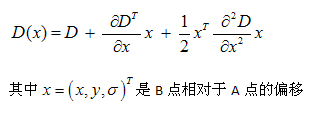求上式极值，对其求导，导数等于0，得到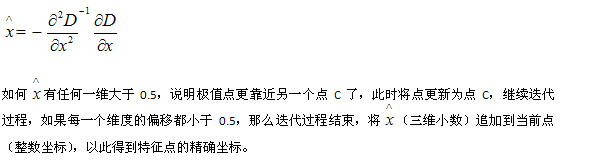去除不稳定特征点
去除对比度低的点
以上求出了极值点的精确的位置，将求出的 x 带入原式，得：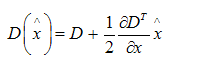我们就利用这个函数去除对比度低的点，lowe文中，当D（x）<=0.03时，去除这个特征点。
去除边缘点
差分高斯金字塔中的极值点会有许多边缘点，边缘点对一些噪声不稳定，因此需要去除这些边缘相应点。
差分高斯金字塔中会有一些不是很好的极值点，这些点的特征是：在跨越边缘的方向有较大的主曲率，在与边缘相切的方向主曲率较小。在本步骤中，需要去除这些不好的边缘相应。主曲率可以通过2阶Hessian方阵获得：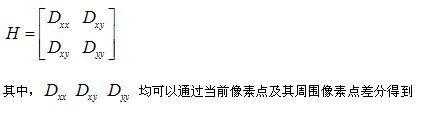D函数中某点的主曲率和该点的H矩阵的特征值是成比例的，因此我们可以通过H矩阵的特征值来确定某点在差分高斯金字塔中的主曲率。
设矩阵H的特征值分别为α（较大）和β（较小），有如下公式：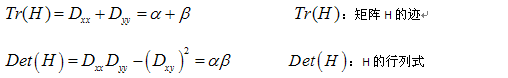通过以上两式，α和β就可以计算出来了，但是，不急！
如上文所述，那些不好的边缘点：跨越边缘的方向有较大的主曲率，与边缘相切的方向主曲率较小。因此，我们通过α/β的比率函数并确定阈值来体现表征那些不好的边缘点，α/β越大，说明这个点就越糟糕，就越应该被删掉，但是这样就要真真切切计算α和β的值，前面让大家不急了，是的，先不用着急计算，设定r=α/β(即 α=rβ)，使用如下公式：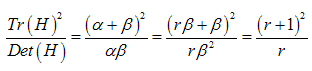以上函数是关于r的增函数（已经假设α是特征值中较大的一个），r 越大，以上函数值就越大，反之，以上函数值越大，r 就是越大的，因此我们可以通过已知的Tr（H）和Det（H）“曲线地”去判断 r的大小！所以在本步骤中，去除不好的边缘点的阈值是：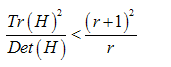lowe论文中设定r=10。
到这里，在差分高斯金字塔中提取的特征点就完成了提纯的步骤。

// Interpolates a scale-space extremum's location and scale to subpixel
// accuracy to form an image feature. Rejects features with low contrast.
// Based on Section 4 of Lowe's paper.
static bool adjustLocalExtrema( const vector<Mat>& dog_pyr, KeyPoint& kpt, int octv,
int& layer, int& r, int& c, int nOctaveLayers,
float contrastThreshold, float edgeThreshold, float sigma )
{
const float img_scale = 1.f/(255*SIFT_FIXPT_SCALE);
const float deriv_scale = img_scale*0.5f;
const float second_deriv_scale = img_scale;
const float cross_deriv_scale = img_scale*0.25f;

float xi=0, xr=0, xc=0, contr=0;
int i = 0;

// 如上文所述，迭代计算特征点的精确位置
for( ; i < SIFT_MAX_INTERP_STEPS; i++ )
{
int idx = octv*(nOctaveLayers+2) + layer;
const Mat& img = dog_pyr[idx];
const Mat& prev = dog_pyr[idx-1];
const Mat& next = dog_pyr[idx+1];

Vec3f dD((img.at<sift_wt>(r, c+1) - img.at<sift_wt>(r, c-1))*deriv_scale,
(img.at<sift_wt>(r+1, c) - img.at<sift_wt>(r-1, c))*deriv_scale,
(next.at<sift_wt>(r, c) - prev.at<sift_wt>(r, c))*deriv_scale);

float v2 = (float)img.at<sift_wt>(r, c)*2;
float dxx = (img.at<sift_wt>(r, c+1) + img.at<sift_wt>(r, c-1) - v2)*second_deriv_scale;
float dyy = (img.at<sift_wt>(r+1, c) + img.at<sift_wt>(r-1, c) - v2)*second_deriv_scale;
float dss = (next.at<sift_wt>(r, c) + prev.at<sift_wt>(r, c) - v2)*second_deriv_scale;
float dxy = (img.at<sift_wt>(r+1, c+1) - img.at<sift_wt>(r+1, c-1) -
img.at<sift_wt>(r-1, c+1) + img.at<sift_wt>(r-1, c-1))*cross_deriv_scale;
float dxs = (next.at<sift_wt>(r, c+1) - next.at<sift_wt>(r, c-1) -
prev.at<sift_wt>(r, c+1) + prev.at<sift_wt>(r, c-1))*cross_deriv_scale;
float dys = (next.at<sift_wt>(r+1, c) - next.at<sift_wt>(r-1, c) -
prev.at<sift_wt>(r+1, c) + prev.at<sift_wt>(r-1, c))*cross_deriv_scale;

Matx33f H(dxx, dxy, dxs,
dxy, dyy, dys,
dxs, dys, dss);//通过当前像素点以及周围像素点差值出H矩阵

Vec3f X = H.solve(dD, DECOMP_LU);

xi = -X;
xr = -X;
xc = -X;

//有任何一个维度的偏移超过0.5，会更新当前像素点
//如果每一个维度的偏移都没有超过0.5,当前像素的位置加上偏移就是最终的精确点
if( std::abs(xi) < 0.5f && std::abs(xr) < 0.5f && std::abs(xc) < 0.5f )
break;

if( std::abs(xi) > (float)(INT_MAX/3) ||
std::abs(xr) > (float)(INT_MAX/3) ||
std::abs(xc) > (float)(INT_MAX/3) )
return false;

c += cvRound(xc);
r += cvRound(xr);
layer += cvRound(xi);

if( layer < 1 || layer > nOctaveLayers ||
c < SIFT_IMG_BORDER || c >= img.cols - SIFT_IMG_BORDER  ||
r < SIFT_IMG_BORDER || r >= img.rows - SIFT_IMG_BORDER )
return false;
}
//迭代结束
// ensure convergence of interpolation
if( i >= SIFT_MAX_INTERP_STEPS )
return false;

{
int idx = octv*(nOctaveLayers+2) + layer;
const Mat& img = dog_pyr[idx];
const Mat& prev = dog_pyr[idx-1];
const Mat& next = dog_pyr[idx+1];
Matx31f dD((img.at<sift_wt>(r, c+1) - img.at<sift_wt>(r, c-1))*deriv_scale,
(img.at<sift_wt>(r+1, c) - img.at<sift_wt>(r-1, c))*deriv_scale,
(next.at<sift_wt>(r, c) - prev.at<sift_wt>(r, c))*deriv_scale);
float t = dD.dot(Matx31f(xc, xr, xi));

contr = img.at<sift_wt>(r, c)*img_scale + t * 0.5f;
if( std::abs( contr ) * nOctaveLayers < contrastThreshold )//去除低对比度的点
return false;

// principal curvatures are computed using the trace and det of Hessian
float v2 = img.at<sift_wt>(r, c)*2.f;
float dxx = (img.at<sift_wt>(r, c+1) + img.at<sift_wt>(r, c-1) - v2)*second_deriv_scale;
float dyy = (img.at<sift_wt>(r+1, c) + img.at<sift_wt>(r-1, c) - v2)*second_deriv_scale;
float dxy = (img.at<sift_wt>(r+1, c+1) - img.at<sift_wt>(r+1, c-1) -
img.at<sift_wt>(r-1, c+1) + img.at<sift_wt>(r-1, c-1)) * cross_deriv_scale;
float tr = dxx + dyy;
float det = dxx * dyy - dxy * dxy;

if( det <= 0 || tr*tr*edgeThreshold >= (edgeThreshold + 1)*(edgeThreshold + 1)*det )//去除边缘噪声点
return false;
}

kpt.pt.x = (c + xc) * (1 << octv);
kpt.pt.y = (r + xr) * (1 << octv);
kpt.octave = octv + (layer << 8) + (cvRound((xi + 0.5)*255) << 16);
kpt.size = sigma*powf(2.f, (layer + xi) / nOctaveLayers)*(1 << octv)*2;
kpt.response = std::abs(contr);

return true;
}

以上SIFT源码均摘自OpenCV nonfree模块，lowe对SIFT拥有版权。
符合要求的特征点构建完毕，需要对该特征点进行描述了，请关注本博客SIFT系列的下一篇文章：SIFT解析（三）生成特征描述子

（转载请注明作者和出处 ：honpey   http://blog.csdn.net/wendy709468104/article/details/8639634 ）


展开全文OpenCV SIFT
最近做快递上门取件业务，当获取用户位置经纬度时，如何快递查询出离用户最近的站点位置，以便让离用户最近的快递小哥上门取件，我的方式是直接在sql语句中计算最近的点
SELECT id,code,name,city_name,address,
FROM bmz_site ORDER BY distance LIMIT 0,10;

/*北京大成39.8995965929,116.4919853210 上海宝地31.2433336586,121.4579772949*/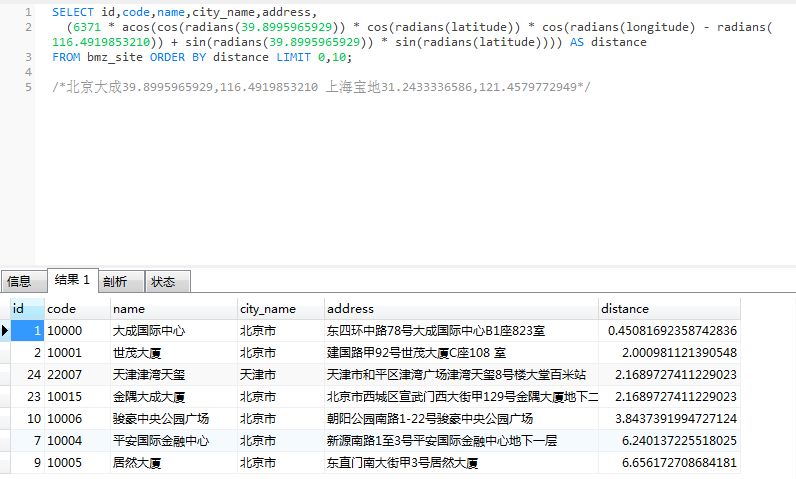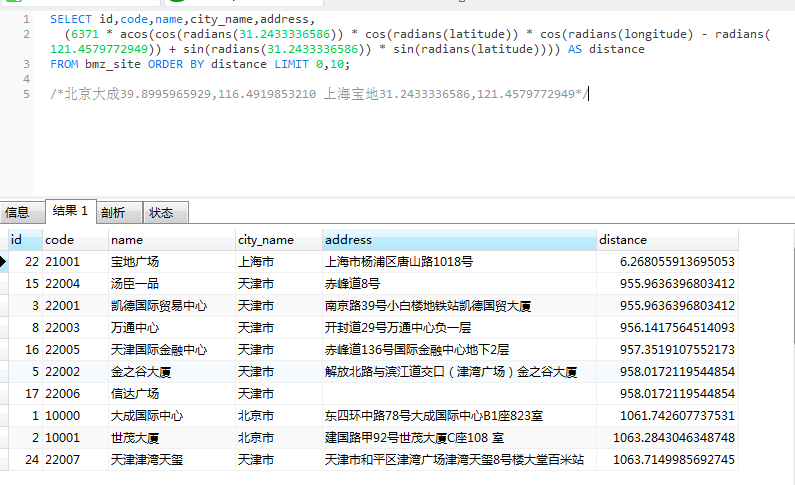我们改变经纬度的值，可以获取离我们的经纬度最近的前十条数据
展开全文• 编写一个矩形类Rect，继承Rect类编写一个具有确定位置的矩形类PlainRect，编写PlainRect类的测试程序 按要求编写一个Java应用程序： （1）编写一个矩形类Rect，包含： 两个protected属性：矩形的宽width；矩形的...
编写一个矩形类Rect，继承Rect类编写一个具有确定位置的矩形类PlainRect，编写PlainRect类的测试程序
按要求编写一个Java应用程序： （1）编写一个矩形类Rect，包含： 两个protected属性：矩形的宽width；矩形的高height。 两个构造方法： 1．一个带有两个参数的构造方法，用于将width和height属性初化； 2．一个不带参数的构造方法，将矩形初始化为宽和高都为10。
两个方法： 求矩形面积的方法area() 求矩形周长的方法perimeter()
（2）通过继承Rect类编写一个具有确定位置的矩形类PlainRect，其确定位置用矩形的左上角坐标来标识，包含： 添加两个属性：矩形左上角坐标startX和startY。
两个构造方法： 带4个参数的构造方法，用于对startX、startY、width和height属性 初始化； 不带参数的构造方法，将矩形初始化为左上角坐标、长和宽都为0 的矩形； 添加一个方法：判断某个点是否在矩形内部的方法isInside(double x,double y)。如在矩形内，返回true, 否则，返回false。
提示：点在矩形类是指满足条件： x>=startX&&x<=(startX+width)&&y<startY&&y>=(startY-height)
（3）编写PlainRect类的测试程序 创建一个左上角坐标为（10，10），长为20，宽为10的矩形对象； 计算并打印输出矩形的面积和周长； 判断点(25.5，13)是否在矩形内，并打印输出相关信息。
Rect.java 👇
package work.eighth;

public class Rect {
protected double width;
protected double height;

public Rect(double width, double height) {
this.width = width;
this.height = height;
}

public Rect() {
width=10;
height=10;
}
public double area(){
return width*height;
}
public double perimeter(){
return (width+height)*2;
}
}


PlainRect.java 👇
package work.eighth;

public class PlainRect extends Rect{
private double startX;
private double startY;

public PlainRect(double width, double height, double startX, double startY) {
super(width, height);
this.startX = startX;
this.startY = startY;
}

public PlainRect() {
super();
startX=0;
startY=0;
}
public boolean isInside(double x,double y){
return (x>=startX&&x<=(startX+width)&&y<startY&&y>=(startY-height));
}
}

PlainRectTest.java 👇
package work.eighth;
public class PlainRectTest {
public static void main(String[] args) {
PlainRect p1 = new PlainRect(20,10,10,10);
System.out.println("面积为："+p1.area());
System.out.println("周长为："+p1.perimeter());
System.out.println(p1.isInside(25.5,13));
}
}


展开全文• 三角化求特征的空间位置


视觉SLAM笔记（35） 三角化求特征点的空间位置

1. 特征点的空间位置
2. 三角测量函数
3. 求特征点的空间位置

1. 特征点的空间位置
在 视觉SLAM笔记（31） 特征提取和匹配 已求出特征点 在 视觉SLAM笔记（33） 对极约束求解相机运动 已根据特征点的对极几何估算出相机的位姿 现在通过三角化求出特征点的空间位置

2. 三角测量函数
创建/VSLAM_note/035/triangulation.cpp文件 其中 特征提取函数 find_feature_matches 与 视觉SLAM笔记（33） 对极约束求解相机运动 相同 2D-2D姿态估计函数 pose_estimation_2d2d 与 视觉SLAM笔记（33） 对极约束求解相机运动 相似
展开全文• 图像中，已知了某连通域的一个像素，如何根据该像素点确定像素所在的连通域(比如图像中有多个连通域，而现在只知一个连通域的像素，如何根据该反推像素所在的连通域，并标记出来)图像处理 算法
• =10000，全部由字母组成)中找到第一个只出现一次的字符,并返回它的位置, 如果没有则返回 -1（需要区分大小写） 思路分析 本题可以先用字典记录每个字符的次数，然后遍历字典，但是考虑需要每次判断字符是否是字典中...字符串
• 这个问题很有趣,首先我第一个直觉是用图论的知识来解决,但是这是不可行的.因为我们没有办法将每一个节点的信息保存起来(这样的代价过于巨大,每一个节点标志唯一的就是它本身的地址,但是地址这个信息不能直接映射为...
• 根据坐标点位置计算方位角是在GPS轨迹处理和...（x1，y1）为当前GPS点坐标，（x2，y2）为下一个点的GPS坐标： def calc_angle(x1,y1,x2,y2): angle=0 dy= y2-y1 dx= x2-x1 if dx==0 and dy&amp;gt;0: ...方位角
• 工作中需要判断与矢量的位置关系，即在矢量左侧或者右侧，找了看了一下理论，其实就是简单的高中知识，只不过都忘差不多了，不过不难，直接上代码（JAVA）。package cn.xue.Algorithm; public class ...
• 如图所示，线段AB和P印象里，只学过三维向量的叉乘，将向量AB和AP扩展到三维，得到跟预计的是相符合的，是一个平行于Z轴的向量，即如果 res&lt;0，说明结果向量垂直于屏幕向里，也就是指向Z轴的负方向，P在...
• 在识别到目标后，有一个很重要的问题：我们的最终目的是瞄准、跟踪、打击，怎样利用识别到目标后得到的目标在图像中的像素坐标来确定在真实世界中目标的位置呢？更清楚说就是我识别得到的是图像中点的坐标，而我要...角度测量
• 理想很丰满，现实很骨感，常常以为实现一个算法不是很难，但在现实中场景更加复杂，...下面介绍一个OpenCV的小接口，用来判断和contour的位置关系 def pointPolygonTest(contour, pt, measureDist): # real sign...python 人工智能
• 在多区域mutiPolygon中位置(高效判断) 问题1.怎样快速判断在某个区域中？ 首先1.在指定多区域Polygon中填充geohash【地理空间索引编码】值，例如指定不规则多边形的区域A （Polygon）填充geohash...地理信息计算
• 实验：熟悉ArcGIS中空间分析工具 ——2012210422陈洪磊 、实验目的 温习和熟悉ArcGIS中空间数据分析的基本流程、基本工具、基本内容及操作步骤，理解地理空间数据的基本特征，会初步分析空间分析方法含义和提取...ArcGIS 距离分析 缓冲分析
• %B是矩阵，5是要查找的元素值，返回行位置m和列位置n 2. 字符型/cell型 查找某个字符串在cell中的位置：m = find(strcmp(Strcell, str1)); %Strcell是cell数组，str1是要查找的字符串，返回位置m ...matlab
• 在软件视图右上角的查询功能区中选择“坐标”功能项，然后输入坐标并点击“查询”按钮后，会在视图中显示一个红色图标标注当前坐标所在位置
• 页面上有两颗星星图标，画条线连接两颗星星。 1.先获取两的坐标 2.计算弦长及旋转角度 3.绘制线 <div class="quality-analysis"> <div class="cnt-box"> <span class="selected-start"> ...
• 不同A,B确定一条直线，AB相同返回的值全0 直线方程：Ax+By+c=0 A = y2 - y1; B = x1 - x2; C = -Ax1 - By1 = x2y1 - x1y2; 证明之后补上； Line LineMake(Point A, Point B) { Line l; l.A = B.y - A.y; l.B...
• 用sub2ind >> a=zeros(5); i = [2;3;4]; j = [1;4;2]; >> a(sub2ind(size(a), i, j))=1 a = 0 0 0 0 0 1 0 0 0 0 0 0 0 1 0 0 1 0 0 0 0 0 0 0 0
• 我们以驾车为例，计算两点之间最优的路线，主要就是从一个点出发，开始找路，首先你可以匹配离你最近的道路，然后出发，在每遇到一个岔路口的时候，都分别去找路，目的就是一直找到可以到达终点的线路。 在这里有的...路线规划 地图
• 之前有一个需求，是要把一些坐标的相对位置画到一张图片上，标注出点，点名，并且之间两两连线生成一张图片。 最开始考虑用html5实现。 但实际上我们生成的图片要在后台放到word文档中去，所以最后使用的php在...html5 html绘图 php php绘图
• //判断与多边形的位置关系 //若在多变形内部，返回-1；若在多变形边界上，返回 0；若在多变形外部，返回 1； //该算法不仅和射线算法有相同的效率，而且对射线算法中特殊情况的处理近乎完美，也很好的避开...算法
• STC学习【1】STC单片机引脚起始位置(一个/两个/三个原点)
• Point Util::Rotate(Point p, double angle, double x, double y, double z) //注意这里的（x,y,z）是单位化的旋转轴向量 {  double m;  //D3DXVec3Normalize();  //angle = 1.57;  double u = x;...
• #include #include using namespace std; int main(int argc, char** argv) { const char* pcExePath = argv; cout ; const char* pcLast = strrchr(pcExePath, '\\');
• 问题描述：判断二维平面上一个点P是在三角形ABC的内部还是外部。 前辈们给我们总结了许多办法，这里我给出一个比较直观也比较简单的办法。 在讲这个办法之前，我们先看另一个问题。 如果给定一条线段AB和点C，...
• 但是这样自动布局带来的后果是很难知道窗口中某部件在主窗口中的相对位置。 在处理子窗口鼠标事件时变的很麻烦。主窗口有菜单、工具条等，想用鼠标绘图， 把鼠标轨迹映射到窗口部件上，这些问题。 ...
• 在工作中经常遇到判断一个点在另一个点的那个方位的问题，如下图，这里需要确定p2在p1的那个方位，也就是求p2相对于p1落在区域1，2，3，4那个中，注意此处p1不是坐标原点，坐标原点在屏幕的左上角（此处为屏幕坐标）...float 算法
• 从该出发,作任意方向的根射线, 考察此射线与三维物体各面的交点数, 如果总数=0或其它偶数,则在三维物体之外, 如果总数为奇,则在三维物体之内. 为了减少时间，如果位置很有可能在三维物体之外时，你最好...流体模拟...